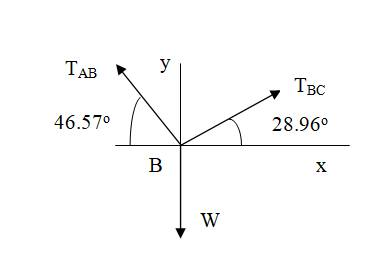# Cable ABC of length 5 m supports the force W at B . Determine (a) the angles β 1 and β 2 ; and (b) the force in each cable segment in terms of W .### International Edition---engineerin...

4th Edition
Andrew Pytel And Jaan Kiusalaas
Publisher: CENGAGE L
ISBN: 9781305501607

#### Solutions

Chapter
Section### International Edition---engineerin...

4th Edition
Andrew Pytel And Jaan Kiusalaas
Publisher: CENGAGE L
ISBN: 9781305501607
Chapter 6, Problem 6.85P
Textbook Problem
3 views

## Cable ABC of length 5 m supports the force W at B. Determine (a) the angles β 1 and β 2 ; and (b) the force in each cable segment in terms of W.To determine

(a)

The angles β1 and β2.

### Explanation of Solution

Given information:

Length of cable ABC is 5 m.

A force W is supported at point B.

Calculations:

From geometry

2cosβ1+3cosβ2=4........................(a)2sinβ13sinβ2=0.         sinβ2=23sinβ1....(b)

Substituting Eq. (b) in the trigonometric identity:

cosβ2=1 sin2β2 we getcosβ2=149 sin2β1=149( 1 cos 2 β 1 )=135+4 cos2β1

Substituting in Eq

To determine

(b)

The force in each cable segment in terms of W.

### Still sussing out bartleby?

Check out a sample textbook solution.

See a sample solution

#### The Solution to Your Study Problems

Bartleby provides explanations to thousands of textbook problems written by our experts, many with advanced degrees!

Get Started

Find more solutions based on key concepts
What is content streaming?

Fundamentals of Information Systems

Describe three systems development tools and three development methods.

Systems Analysis and Design (Shelly Cashman Series) (MindTap Course List)

What is PCI DSS and why is it important for information security?

Principles of Information Security (MindTap Course List)

Measure the width and the length of a treadmill machine. Discuss what some of the design factors are that deter...

Engineering Fundamentals: An Introduction to Engineering (MindTap Course List)

Explain how ARPANET contributed to the growth of the Internet.

Enhanced Discovering Computers 2017 (Shelly Cashman Series) (MindTap Course List)

Name two types of respirators and describe how they work.

Welding: Principles and Applications (MindTap Course List)

If your motherboard supports ECC DDR3 memory, can you substitute non-ECC DDR3 memory?

A+ Guide to Hardware (Standalone Book) (MindTap Course List)